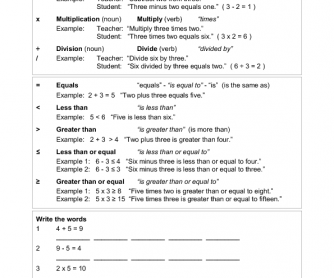Math in English

rated by 7 teachers
by
 This is simple addition, subtraction, multiplication and division and introduces students to using English in doing Math. Introduces the vocabulary 'plus', 'minus', 'times', 'divided by' and 'equals'. Example: Teacher says: "Add three and six." Student says: "Three plus six equals nine." Includes a crossword puzzle to reinforce the lesson. Similar Worksheets Calculator Fun: Math Worksheet Divide Numbers by 1 to 10 Solving the Equation - Multiplicatio ... #attachment {font-size:16px;text-align:center;} .btn-signinnow:hover {color:#fff!important;text-decoration:none!important;background-color:#2F96B4!important;} #attachment a:hover {text-decoration:none!important;width:170px!important;}